# 基于冲激响应原理的步进频率探地雷达信号快速正演模拟及融合Fast Forward Simulation and Fusion for Stepped Frequency Ground Penetrating Radar Signal Based on Impulse Response Theory

DOI: 10.12677/AG.2021.114044, PDF, HTML, XML, 下载: 21  浏览: 44  国家自然科学基金支持

Abstract: Stepped-frequency continuous wave ground penetrating radar (SFCW GPR) is a kind of frequen-cy-domain GPR. It has controllable signal frequency, bigger average transmitting power, deeper penetration and higher resolution compared to pulsed GPR. At present, stepped frequency ground penetrating radar contains different frequencies of transmit signal, so the amount of calculation in the numerical simulation has doubled, and the signal fusion method is not yet mature. This paper conducts forward simulation research of SFCW GPR, the open source program gprMax has been used combined with the impulse response of linear time-invariant system to improve simulation efficiency and avoid repeated forward calculation. The reflected signal obtained by simulation is mixed with transmitted signal and goes through low-pass filter to extract the phase information related to the distance of target, then the result is converted to time domain through inverse Fourier transform for signal fusion. We have compared the anti-noise performance of pulsed GPR and SFCW GPR by adding noise to the simulation. The results show that SFCW GPR has a higher signal-to-noise ratio for target detection under noisy condition.

1. 引言

2. 原理

2.1. SFCW探地雷达基本原理

${f}_{n}={f}_{0}+\left(n-1\right)\Delta f$ (1)

$Tx\left(n\right)=\mathrm{sin}2\pi \left({f}_{0}+\left(n-1\right)\Delta f\right)t$ (2)

$Rx\left(n\right)={A}_{n}\mathrm{sin}2\pi \left({f}_{0}+\left(n-1\right)\Delta f\right)\left(t-\frac{2d}{v}\right)$  (3)

${\varphi }_{n}=2\pi \left({f}_{0}+\left(n-1\right)\Delta f\right)\frac{2d}{v}$ (4)

$Rx\left(n\right)={A}_{n}\mathrm{sin}\left(2\pi {f}_{n}t-{\varphi }_{n}\right)$ (5)

2.2. gprMax正演及LTI系统的冲激响应

gprMax是用python语言编写的探地雷达FDTD正演软件，可用来进行多种电磁问题的数值模拟。其操作采用命令行窗口的形式，需要编写规定格式的模型文件(.in文件)，在模型文件中定义介质的空间分布和电性参数(相对介电常数εr，电导率σ，相对磁导率μr，磁损耗σr)，给出激励源的类型和位置等参数，将模型文件路径输入到gprMax命令行窗口中，即可调用程序进行计算，输出文件为若干道雷达反射数据(.out文件)。利用gprMax进行正演模拟时，首先要对正演模型进行空间离散和时间离散。

$dx/dy/dz\le \frac{c}{{f}_{\mathrm{max}}\sqrt{{\epsilon }_{\mathrm{max}}}}×\frac{1}{10}$ (6)

$dt\le \frac{1}{c\sqrt{\frac{1}{{\left(dx\right)}^{2}}+\frac{1}{{\left(dy\right)}^{2}}+\frac{1}{{\left(dz\right)}^{2}}}}$ (7)Figure 1. Impulse response theory of LTI system

$y\left[n\right]=x\left[n\right]\ast h\left[n\right]={\sum }_{k=-\infty }^{+\infty }x\left[k\right]h\left[n-k\right]$  (8)

3. 模型正演算例

3.1. 二维地质模型构建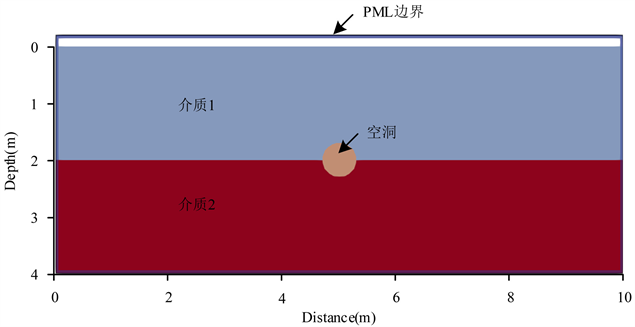Figure 2. 2D geological modelTable 1. Electrical parameters of media in model

3.2. 发射信号生成及反射信号获取

$Tx\left(t\right)=\left\{\begin{array}{l}k{f}_{n}t\mathrm{sin}\left(2\pi {f}_{n}t\right)\text{if}\text{\hspace{0.17em}}k{f}_{n}t<1\\ \mathrm{sin}\left(2\pi {f}_{n}t\right)\text{if}\text{\hspace{0.17em}}k{f}_{n}t\ge 1\end{array}$ (9)Figure 3. Transmit signal of 100 MHz

$\delta \left(t\right)=\left\{\begin{array}{l}1\text{if}\text{\hspace{0.17em}}t (10)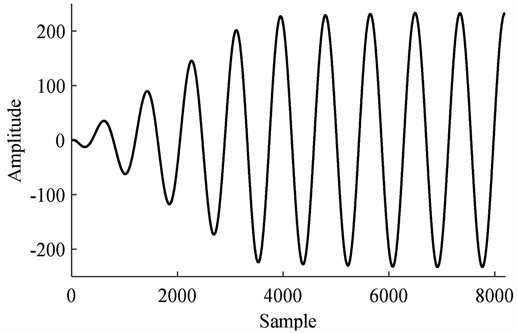Figure 4. Reflected signal from simulation of Figure 3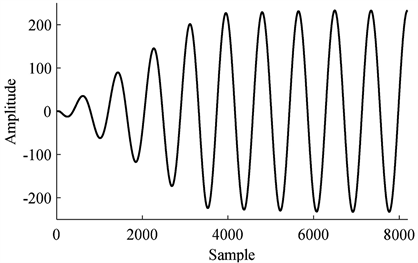Figure 5. Signal from convolution of Figure 3 and impulse response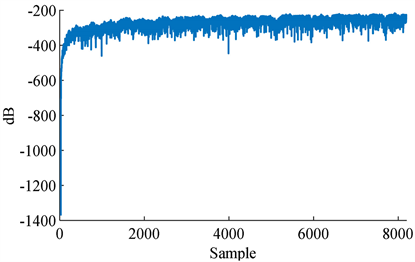Figure 6. dB error of Figure 4 and Figure 5

3.3. 信号处理与融合

SFCW探地雷达正演的流程见图7，通过正演得到反射信号后，需要将得到的反射信号进行正交分解。其中同相分量I和正交分量Q分别由以下公式得到：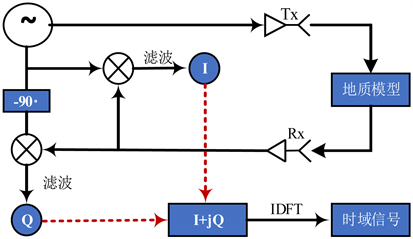Figure 7. Flow of SFCW GPR modeling

${I}_{n}={A}_{n}\mathrm{sin}\left(2\pi {f}_{n}t-{\varphi }_{n}\right)\ast \mathrm{sin}\left(2\pi {f}_{n}t\right)=\frac{{A}_{n}}{2}\left(\mathrm{cos}\left(4\pi {f}_{n}t-{\varphi }_{n}\right)-\mathrm{cos}\left({\varphi }_{n}\right)\right)$ (11)

${Q}_{n}={A}_{n}\mathrm{sin}\left(2\pi {f}_{n}t-{\varphi }_{n}\right)\ast \mathrm{cos}\left(2\pi {f}_{n}t\right)=\frac{{A}_{n}}{2}\left(\mathrm{sin}\left(4\pi {f}_{n}t-{\varphi }_{n}\right)-\mathrm{sin}\left({\varphi }_{n}\right)\right)$ (12)

${I}_{n}=-\frac{{A}_{n}}{2}\mathrm{cos}\left({\varphi }_{n}\right)$ (13)

${Q}_{n}=-\frac{{A}_{n}}{2}\mathrm{sin}\left({\varphi }_{n}\right)$ (14)

$Rx\left(n\right)={I}_{n}+j{Q}_{n}$ (15)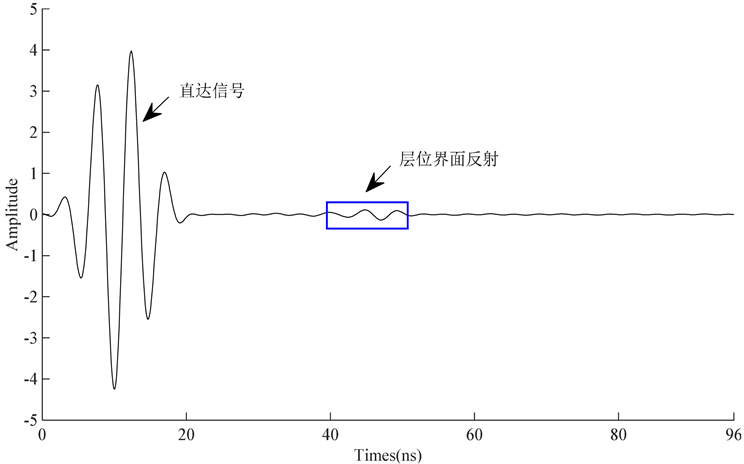Figure 8. The first synthetic signal

4. SFCW和脉冲体制雷达抗噪性能对比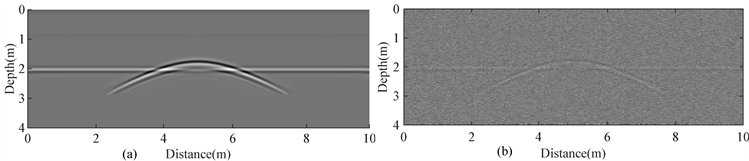Figure 9. The simulation result of Ricker wave signal (a) Result without noise; (b) Result with noise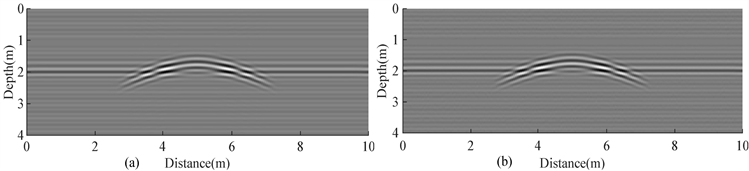Figure 10. The simulation result of SFCW signal (a) Result without noise; (b) Result with noise

5. 结论

1) SFCW信号用于探地雷达探测时，主要是将目标距离信息转化为不同频点连续波的相移，再通过逆傅里叶变换转换到时域内进行信号融合，以实现对目标体的检测；

2) 将SFCW信号进行正演计算时，结合LTI系统的冲激响应，在保证精度的条件下，可以避免重复进行正演计算，提高正演效率；

3) 根据对SFCW信号和Ricker子波脉冲信号的抗噪性能对比研究表明，在同样的噪声条件下，SFCW探地雷达结果具有更高的信噪比，抗噪性能更好。

NOTES

*第一作者。

#通讯作者。

  Xiao, J. and Liu, L. (2015) Permafrost Subgrade Condition Assessment Using Extrapolation by Deterministic Decon-volution on Multifrequency GPR Data Acquired along the Qinghai-Tibet Railway. IEEE Journal of Selected Topics in Applied Earth Observations and Remote Sensing, 9, 83-90.  陈昆, 李跃华, 陈思思. 步进频率雷达回波采样数据处理[J]. 微波学报, 2012(S1): 267-270.  陶春康, 汪海勇, 瞿诗华. 步进扫频宽带探地雷达信号处理[J]. 微波学报, 2020, 6(2): 67-70.  许泽善, 周江涛, 刘四新, 等. 三维步进频率探地雷达在沥青层厚度检测中的应用[J]. 物探与化探, 2019, 43(5): 1145-1150.  Tronca, G., Tsalicoalou, I., Lehner, S., et al. (2018) Comparison of Pulsed and Stepped Frequency Continuous Wave (SFCW) GPR Systems. 2018 17th International Conference on Ground Penetrating Radar (GPR), Rapperswil, 18-21 June 2018, 1-4. https://doi.org/10.1109/ICGPR.2018.8441654  Hamran, S.E., Gjessing, D.T., Hjelmstad, J., et al. (1995) Ground Penetrating Synthetic Pulse Radar: Dynamic Range and Modes of Operation. Journal of Applied Geophysics, 33, 7-14. https://doi.org/10.1016/0926-9851(95)90025-X  Pieraccini, M. (2017) Noise Performance Comparison between Continuous Wave and Stroboscopic Pulse Ground Penetrating Radar. IEEE Geoscience and Remote Sensing Letters, 15, 222-226. https://doi.org/10.1109/LGRS.2017.2781458  Kafedziski, V., Pecov, S. and Tanevski, D. (2018) Target Detection in SFCW Ground Penetrating Radar with C3 Algorithm and Hough Transform Based on gprMax Simulation and Experimental Data. 2018 25th International Conference on Systems, Signals and Image Processing (IWSSIP), Maribor, 20-22 June 2018, 1-5. https://doi.org/10.1109/IWSSIP.2018.8439227  王珣. 探地雷达正演模拟及全波形反演新算法研究[D]: [博士学位论文]. 长沙: 中南大学, 2020.  Yee, K. (1966) Numerical Solution of Initial Boundary Value Problems Involving Maxwell’s Equations in Isotropic Media. IEEE Transactions on Antennas and Propagation, 14, 302-307. https://doi.org/10.1109/TAP.1966.1138693  梁小强, 杨道学, 张可能, 等. FDTD数值模拟在GPR管线探测中的应用[J]. 地球物理学进展, 2017, 32(4): 1803-1807.  冯德山. 地质雷达二维时域有限差分正演[D]: [硕士学位论文]. 长沙: 中南大学, 2003.  Warren, C., Giannopoulos, A. and Giannakis, I. (2016) gprMax: Open Source Software to Simulate Electromagnetic Wave Propagation for Ground Penetrating Radar. Computer Physics Communications, 209, 163-170. https://doi.org/10.1016/j.cpc.2016.08.020  肖都, 李文杰, 郭鹏. 基于GPRMax的隧道衬砌检测数值模拟及应用[J]. 物探与化探, 2015, 39(4): 855-859.  粟毅, 黄春琳, 雷文太. 探地雷达理论与应用[M]. 北京: 科学出版社, 2006: 61-62.  (美)奥本海姆, (美)威尔斯基, (美)纳瓦卜. 信号与系统[M]. 第二版. 刘树棠, 译. 北京: 电子工业出版社, 2013: 48-56.